## Seasonality and Trend Forecast with Regression in Excel

In our last article, we discussed Seasonality in Financial Modeling and Analysis. We went over an example Excel model of calculating a forecast with seasonality indexes.

Today we will use regression analysis in Excel to forecast a data set with both seasonality and trend.

Let’s look at the quarterly sales revenue of the electronic cameras manufacturer GoPro (source: https://www.macrotrends.net/stocks/charts/GPRO/gopro/revenue).

We have the data for the period 2013 to 2019. The aim is to create a model that can help us forecast the revenue of GoPro for the next financial year, 2020. If we add the quarters to the data and plot it on a simple line chart we get:## Is there seasonality?

One way to detect seasonality is by visually examining the data. As soon as we look at the line chart above, we notice some spikes in Q4. Some years show a more prominent jump in sales revenue than others, but we can safely conclude there’s some seasonality in the data series.Another way to test for seasonality is to calculate the average sales revenue for each quarter in the different years. We can calculate these averages with the AVERAGEIFS function in Excel:This approach shows us the same thing. The last quarter of the year is where the company performs best in terms of generating sales revenue.

## Multiple Regression Model

To capture both the seasonality and potential underlying trend in the data, we will rely on the regression analysis functionality that is part of Excel. We are going to treat every quarter as a separate dummy variable. We will have three dummy variables (n-1) for Q1, Q2, and Q3, while Q4 will remain our baseline. The period (t) variable will be the fourth variable in the regression model and will represent our time series.

Keep in mind that if you use specialized statistics software (e.g., R or Python libraries), you won’t have to create the dummy variables, as these frameworks recognize the quarter as a categorical variable. However, if we want to stick to Excel, we need to add these manually.

For the three variables we added for quarters one to three, let’s add a formula to check whether the quarter in each next row matches one of the dummy variables. If they match, we show one (1) in the respective column, and if they don’t, we show zero (0).It’s time to create the model. We will use Excel’s Data Analysis tools (Data Tab > Analyze > Data Analysis). In case you don’t have this visible in your Excel installation, you might have to enable it from the Options menu (File > Options > Add-ins > Excel Add-ins > Analysis ToolPak).

Once you run the Data Analysis tool, select Regression, and click OK to move forward.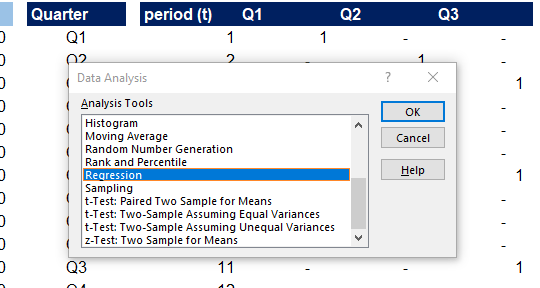The Regression analysis tool opens, and we need to select our Y-Values, which is the dependent variable, or GoPro’s sales revenues for each quarter. We also choose our X-Values, the independent variables. Here we add the period (our time-series variable) and the three dummy variables for Q1, Q2, and Q3. Don’t forget to tick the Labels checkbox if you selected these as well.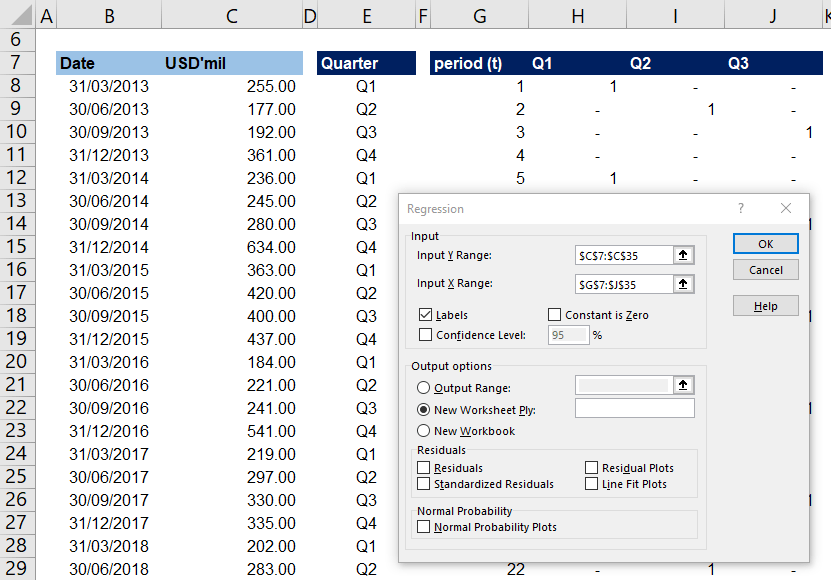## Did We Model Seasonality Well?

Once we generate the regression analysis, we can examine some basic metrics. First, let’s look at the value of R-squared.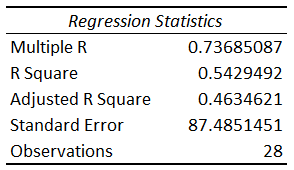Adjusted R-squared varies between 0% and 100% and shows how well the model explains the variability in the data. Generally, we consider models with higher R-squared better, but this is not always the case. For an overall sales forecast (the task at hand), I would find 46% to represent a good-enough fit.

To ensure our model is reliable (statistically significant), we take a look at the ‘Significance F’ metric of our Regression. If it is less than 0.05 (remember, we left our confidence interval at 95%), our model is significant. If it were higher than 0.05, it would’ve meant this set of independent variables wouldn’t give us a good model.Another thing we can look at is the p-values of the independent variables. We won’t go into too much detail on the p-values, as we want to keep this a simple example. Generally, the idea is that most (or ideally all) of those should be below 0.05 (for the same confidence interval of 95%).

You can also see that the coefficients for all variables are negative. This confirms the fact that our baseline (Q4) is where GoPro performs best in terms of sales revenue.We notice that the period (t) variable has a high p-value. This suggests that the period is not significant for our model. If we add a trendline to GoPro’s historical data, we can see that this has been the case for the past few years.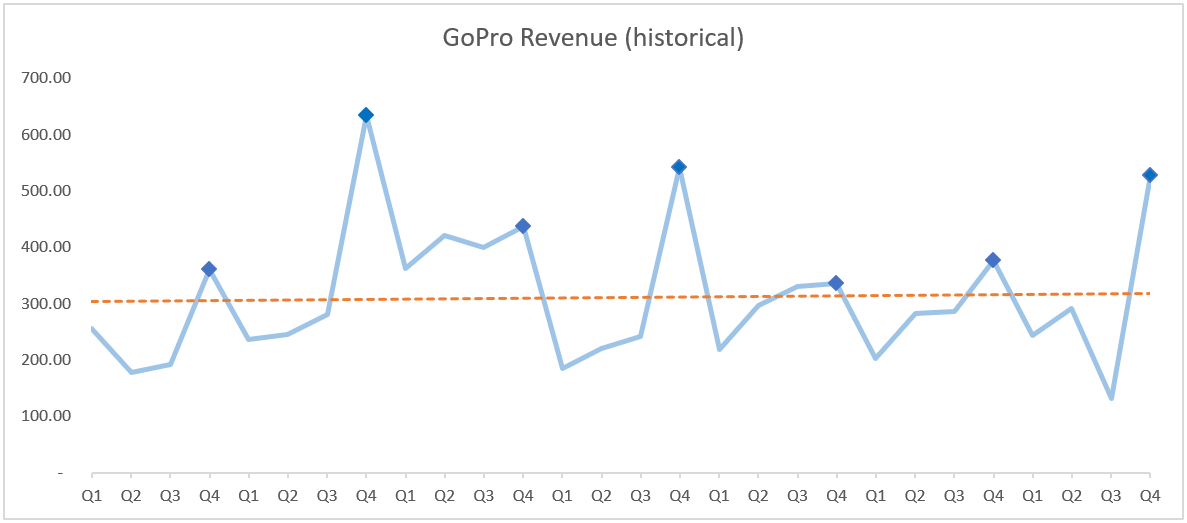Based on our regression metrics review, the model will not be a great predictor of the data set. Here’s the moment where you need to consider the task at hand. For a rough estimation of the future performance of the company, as part of our forecasting efforts, I believe this model to be good enough.

## Forecasting with Seasonality

Let’s calculate the sales performance with our regression model and compare it to the historical data. For each period, the estimated value will equal the intercept of our model plus each of the dummy variables multiplied by their respective coefficients. To achieve this, we can employ the MMULT function in Excel. This gives us the product of each cell from a row range with the corresponding cell from a column range.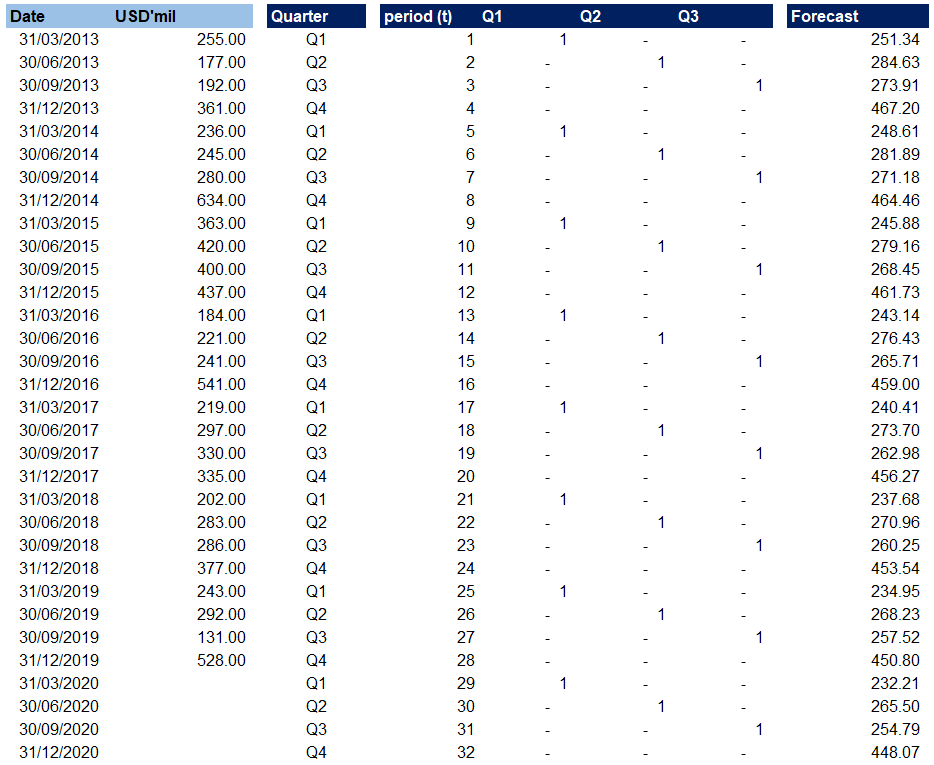Notice that we have extended our forecast to include FY 2020. We can now plot the actual historical values and our projections.## Conclusion

The regression model we prepared for GoPro’s seasonal data does not estimate values that fit the data set completely. However, given the volatility in GoPro’s sales performance over the past few years, I believe this is a sound way to forecast the revenue for the next financial year. It is a usable model which we generated fast and can now use to predict the future sales performance of the company.

Please, show your support by sharing the article with colleagues and friends. Also, don’t forget to download the free Excel example model below.## FCCA, FMVA

Hi! I am a finance professional with 10+ years of experience in audit, controlling, reporting, financial analysis and modeling. I am excited to delve deep into specifics of various industries, where I can identify the best solutions for clients I work with.

In my spare time, I am into skiing, hiking and running. I am also active on Instagram and YouTube, where I try different ways to express my creative side.